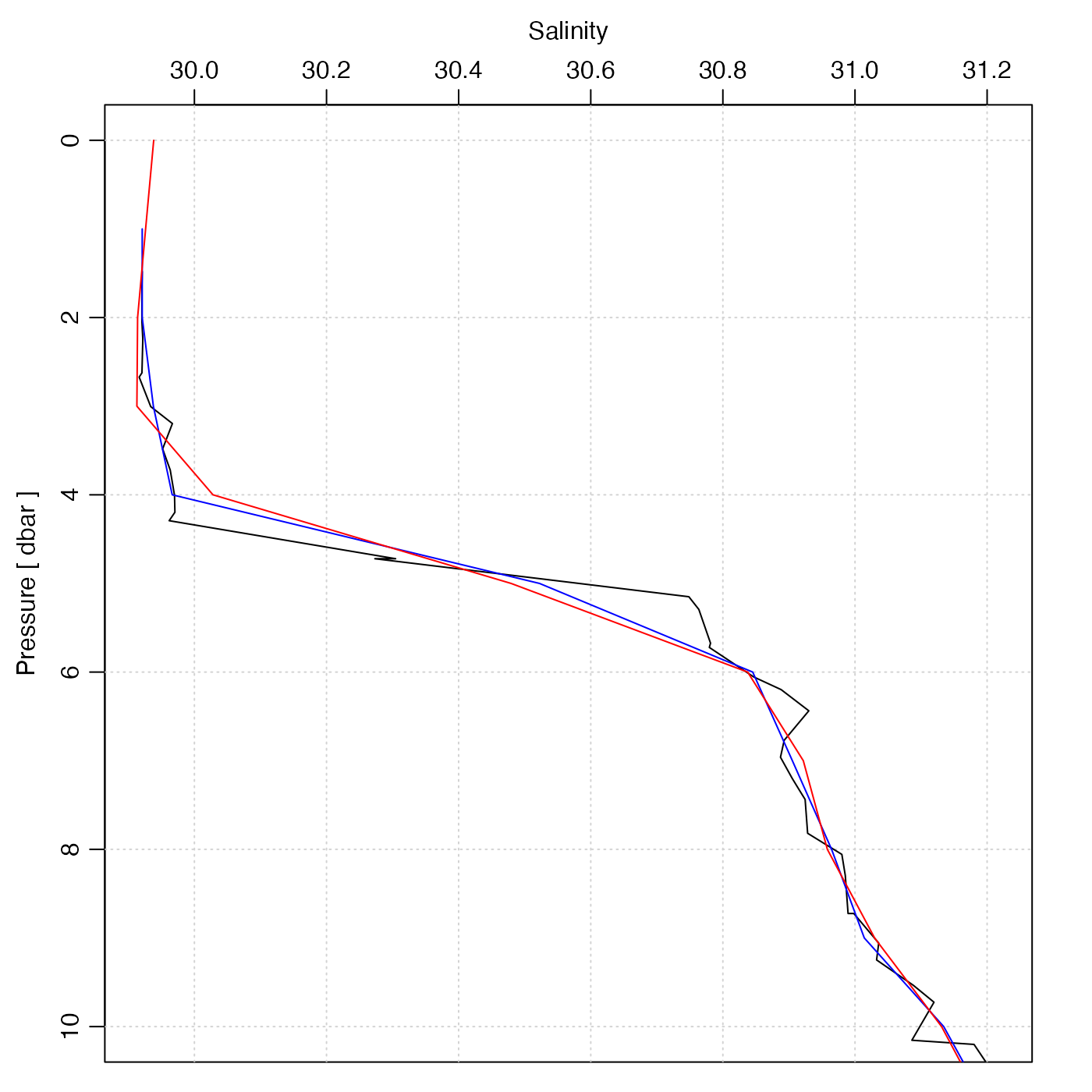Interpolate a CTD profile to specified pressure values. This is used by sectionGrid(), but is also useful for dealing with individual CTD/bottle profiles.

ctdDecimate(
x,
p = 1,
method = "boxcar",
rule = 1,
e = 1.5,
debug = getOption("oceDebug")
)

## Arguments

x

a ctd object.

p

pressure increment, or vector of pressures. In the first case, pressures from 0dbar to the rounded maximum pressure are used, incrementing by p dbars. If a vector of pressures is given, interpolation is done to these pressures.

method

the method to be used for calculating decimated values. This may be a string specifying the method, or a function. In the string case, the possibilities are as follows.

• "boxcar" (based on a local average)

• "approx" (based on linear interpolation between neighboring points, using approx() with the rule argument specified here)

• "approxML" as "approx", except that a mixed layer is assumed to apply above the top data value; this is done by setting the yleft argument to approx(), and by calling that function with rule=c(2, 1))

• "lm" (based on local regression, with e setting the size of the local region);

• "rr" for the Reiniger and Ross method, carried out with oce.approx();

• "unesco" (for the UNESCO method, carried out with oce.approx().

On the other hand, if method is a function, then it must take two arguments, named data and parameters. The first is set to x@data by ctdTrim(). The second is passed directly to the user's function (see Example 2). The return value from the function must be a logical vector of the same length as the pressure data, with TRUE values meaning to keep the corresponding entries of the data slot.

rule

an integer that is passed to approx(), in the case where method is "approx". Note that the default value for rule is 1, which will inhibit extrapolation beyond the observed pressure range. This is a change from the behaviour previous to May 8, 2017, when a rule of 2 was used (without stating so as an argument).

e

is an expansion coefficient used to calculate the local neighbourhoods for the "boxcar" and "lm" methods. If e=1, then the neighbourhood for the i-th pressure extends from the (i-1)-th pressure to the (i+1)-th pressure. At the endpoints it is assumed that the outside bin is of the same pressure range as the first inside bin. For other values of e, the neighbourhood is expanded linearly in each direction. If the "lm" method produces warnings about "prediction from a rank-deficient fit", a larger value of "e" should be used.

debug

an integer specifying whether debugging information is to be printed during the processing. This is a general parameter that is used by many oce functions. Generally, setting debug=0 turns off the printing, while higher values suggest that more information be printed. If one function calls another, it usually reduces the value of debug first, so that a user can often obtain deeper debugging by specifying higher debug values.

## Value

A ctd object, with pressures that are as set by the "p" parameter and all other properties modified appropriately.

## Details

The "approx" and "approxML" methods may be best for bottle data, in which the usual task is to interpolate from a coarse sampling grid to a finer one. The distinction is that "approxML" assumes a mixed-layer above the top sample value. For CTD data, the "boxcar" method may be the preferred choice, because the task is normally to sub-sample, and some degree of smoothing is usually desired. (The "lm" method can be quite slow, and its results may be quite similar to those of the boxcar method.)

For widely-spaced data, a sort of numerical cabeling effect can result when density is computed based on interpolated salinity and temperature. See reference 2 for a discussion of this issue and possible solutions.

Data-quality flags contained within the original object are ignored by this function, and the returned value contains no such flags. This is because such flags represent an assessment of the original data, not of quantities derived from those data. This function produces a warning to this effect. The recommended practice is to use handleFlags() or some other means to deal with flags before calling the present function.

The documentation for ctd explains the structure of CTD objects, and also outlines the other functions dealing with them.

Other things related to ctd data: CTD_BCD2014666_008_1_DN.ODF.gz, [[,ctd-method, [[<-,ctd-method, as.ctd(), cnvName2oceName(), ctd-class, ctd.cnv.gz, ctdFindProfilesRBR(), ctdFindProfiles(), ctdRaw, ctdRepair(), ctdTrim(), ctd_aml.csv.gz, ctd, d200321-001.ctd.gz, d201211_0011.cnv.gz, handleFlags,ctd-method, initialize,ctd-method, initializeFlagScheme,ctd-method, oceNames2whpNames(), oceUnits2whpUnits(), plot,ctd-method, plotProfile(), plotScan(), plotTS(), read.ctd.aml(), read.ctd.itp(), read.ctd.odf(), read.ctd.odv(), read.ctd.saiv(), read.ctd.sbe(), read.ctd.ssda(), read.ctd.woce.other(), read.ctd.woce(), read.ctd(), setFlags,ctd-method, subset,ctd-method, summary,ctd-method, woceNames2oceNames(), woceUnit2oceUnit(), write.ctd()

Dan Kelley

## Examples

library(oce)
data(ctd)
plotProfile(ctd, "salinity", ylim=c(10, 0))
p <- seq(0, 45, 1)
ctd2 <- ctdDecimate(ctd, p=p)
lines(ctd2[["salinity"]], ctd2[["pressure"]], col="blue")
p <- seq(0, 45, 1)
ctd3 <- ctdDecimate(ctd, p=p, method=function(x, y, xout)
predict(smooth.spline(x, y, df=30), xout)\$y)
lines(ctd3[["salinity"]], ctd3[["pressure"]], col="red")